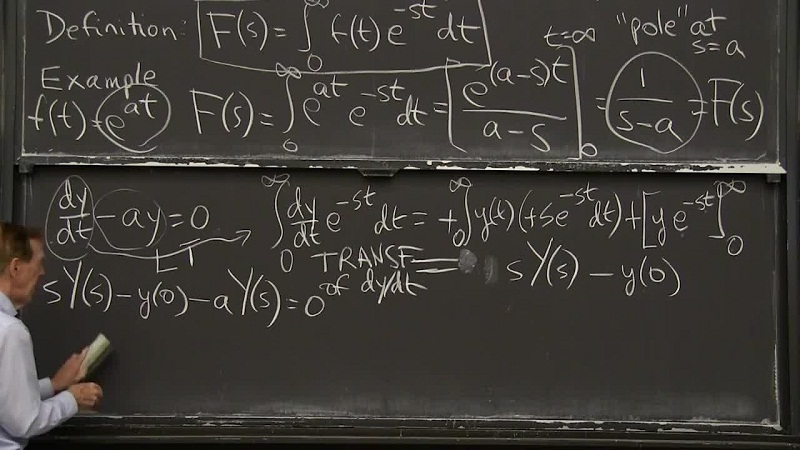Linear Algebra and Partial Differential Equations - MA8352

Online Study Material, Lecturing Notes, Assignment, Reference, Wiki and important questions and answersFOURIER SERIES SOLUTIONS OF PARTIAL DIFFERENTIAL EQUATIONS

MA8352 Linear Algebra and Partial Differential Equations - Anna University 2017 Regulation Syllabus - Download Pdf
MA8352 Linear Algebra and Partial Differential Equations - Question Bank - Download Pdf